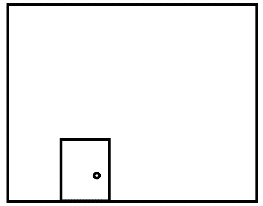# Ex.11.1 Q8 Perimeter and Area - NCERT Maths Class 7

Go back to  'Ex.11.1'

## Question

A door of length $$2\,\rm{}m$$ and breadth $$1\,\rm{}m$$ is fitted in a wall. The length of the wall is $$4.5\,\rm{}m$$ and the breadth is $$3.6\,\rm{}m$$ (See the below Fig). Find the cost of white washing the wall, if the rate of white washing the wall is $$\rm{}Rs\, 20 \,per\, m^2$$Fig 11.6.

Video Solution
Perimeter And Area
Ex 11.1 | Question 8

## Text Solution

What is known?

A door of length $$2\,\rm{}m$$ and breadth $$1\,\rm{}m$$ is fitted in a wall of length $$4.5\,\rm{}m$$ and the breadth $$3.6\,\rm{}m$$

What is unknown?

The cost of white washing the wall, if the rate of white washing the wall is $$\rm{}Rs\, 20 \,per\, m^2.$$

Reasoning:

Since door will not be whitewashed, we will have to subtract area of the door from the area of wall. After, finding area to be whitewashed, multiply the area with rate of white washing $$\rm{}per\, m^2$$ to get the cost.

Steps:

Given,

Length of wall $$= 4.5\,\rm{}m$$

Breadth of wall $$= 3.6\,\rm{}m$$

\begin{align}\text{Area of wall}&= {\rm{Length}} \times {\rm{Breadth}}\\ &= 4.5 \times 3.6{\rm{ }}\\&= 16.2{\rm{ }}{\rm \,m^2}\end{align}

Given,

Length of door $$= 2\,\rm{}m$$

Breadth of door $$= 1\,\rm{}m$$

So,

\begin{align}\text{Area of door}&= {\rm{Length}} \times {\rm{Breadth}}\\&= 2 \times 1\\&= 2{\rm{ }}{\rm \,m^2}\end{align}

\begin{align}&\text{Area of wall for white wash}\\ &= {\text{Area of wall}} - {\text{Area of door}} \end{align}

\begin{align} &= 16.2{\rm{ }}{\rm \,m^2} - 2{\rm{ }}{\rm \,m^2}\\&= 14.2{\rm{ }}{\rm \,m^2}\end{align}

The rate of white washing the wall

$$= \rm{Rs }\,20\,{\rm{ per\, }}{\rm m^2}$$

Therefore,

\begin{align}{\text{The cost of white washing}}\;1618{\rm{ }}{\,\rm m^2} \end{align}

\begin{align} &= 14.2 \times 20\\ &= {\rm{Rs\, }}284\end{align}

Learn from the best math teachers and top your exams

• Live one on one classroom and doubt clearing
• Practice worksheets in and after class for conceptual clarity
• Personalized curriculum to keep up with school